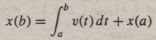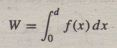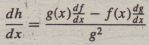Review of Integration and Differentiation

The integral of a function f(x) for a ≤ x ≤ b can be interpreted as the area between the f(x) curve and the x-axis, bounded by the limits x = a and x = b. Figure 8.1-1 illustrates this area. If we denote this area by A, then we can write A asThe integral is f(x):The lower and upper limits of integration here are a and b. the symbol x here is the variable of integration.
Integrals are often encountered in engineering applications. Here are some
examples:
Acceleration and velocity: An object’s velocity v(b) at time t = b, starting with velocity v(0) at t =0í is the integral of its acceleration a(t):Velocity and distance: The position of an object moving at velocity v(t) from the time t = a to the time t = b and starting at position x(a) at t = a isCapacitor voltage and current: The charge Q across a capacitor is the integral of the current i applied to the capacitor. If a capacitor initially holds a charge Q(a), then if the current i(t) is applied from time t =a to time t = b, the capacitor voltage v- iswhere C is the capacitance of the capacitor.

Work expended: The mechanical work done in pushing an object a distance d is the integralwhere ƒ (x) is the force as a function of position x. For a linear spring ƒ(x) =kx, where k is the spring constant, and the work done against the spring ,isIn many applications one or both integral limits are variables. For example, an object’s velocity at time t is the integral of its acceleration. If the object is moving at time t = 0 with a velocity v(0),its velocity is given byIntegrals have the linearity property. If c and d are not functions of x, thenAnother useful property of integrals is the following:The integrals of many functions can be evaluated analytically. We can obtain a formula for the integral, and we are said to have obtained the answer in “closed form.” Here are some common examples that .you have probably seen before:The integrals we have seen thus far are called definite integrals because they have specified limits of integration. Indefinite integrals do not have the limits specified. For example, the following integral is indefinite:Not every function can be integrated analytically. For example, the following is Fresnel’s cosine integral. which has not been evaluated analytically:In such cases we must compute the definite integral using numerical methods.
These are treated in Section 8.2.

Improper Integrals and Singularities

Some integrals have infinite values, depending on their integration limits. These
are called improper integrals. For example, the following integral can be found
in most integral tables:However, it is an improper integral if the integration limits include the point x = 1. To see why, consider Figure 8.1-2, which is a plot of the function y = 1/(x – 1). The function becomes undefined as x approaches 1 from the left or the right. To show that the integral is improper, take the limit of the integral as the upper integration limit h approaches 1 from the left.because In 0 = -∞.So even though an integral can be found in an integral table, you should examine the integrand to check for points at which the integrand is undefined. These points are called singularities. If singularities lie on or within the integration limits, you need to check the limit of the integral to see whether it

is improper: The same warning applies when using numerical methods to evaluate integrals. The points at which the integrand is undefined can cause problems for the numerical method.

Derivatives

Differentiation and integration are complementary operations. For example, ifthen f (x) is the derivative of g(x) with respect to x. This relation is written asThe derivative of ƒ(x) can be interpreted geometrically as the slope of f (x). Thus the derivative is sometimes called the “slope function.
A few basic rules and a short table of derivatives enable us to compute derivatives
of complicated functions. The product rule says that if h (x) = ƒ(x )g(x), thenThe quotient rule states that if h (x) = ƒ(x)/ g(x), thenThe following chain rule enables us to obtain derivatives by decomposing functions into basic functions whose derivatives are given in a table. If z = f(y) and if y = g(x), then z is indirectly a function of x, and the chain rule says thatHere are a few examples of derivatives:Using this short table of derivatives and the product rule, we can show thatUse the chain rule with z = y 2 and y = sin x to obtain the following derivative: